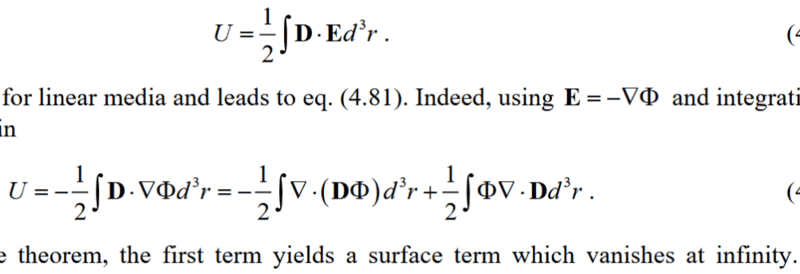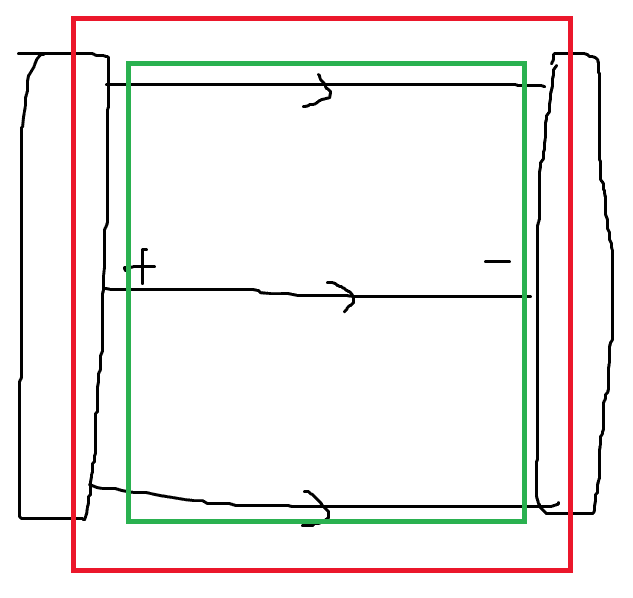# Electrostatic energy in finite space

• feynman1
In summary, the surface integral of a capacitor vanishes if you integrate over the finite volume of the capacitor. The first volume integral of a capacitor will yield a surface integral that contributes to the energy of the capacitor.f

#### feynman1

Electrostatic energy involves a volume integral and a surface integralThe question is how to apply this formula to a finite space in which case the 1st term (surface integral) won't vanish. Let's apply to a capacitor and enclose the capacitor by a closed surface. Calculate the energy integral in this closed volume, then the first surface integral does contribute. How to understand the contribution of the nonzero first surface integral term?

If the electric displacement field lines stop at some point in space, there must be a free surface charge density to stop them. It takes energy to assemble the free charges at the finite boundary.

If the electric displacement field lines stop at some point in space, there must be a free surface charge density to stop them. It takes energy to assemble the free charges at the finite boundary.
Thanks a lot for your reply, however, As is known to all the energy of a capacitor is only restricted to the region between the two parallel plates, just the contribution of the second volume integral. Then how will the first surface integral contribute and how to explain it?

The surface integral vanishes if you integrate over the finite volume of capacitor because D is zero outside the capacitor.

In the case of the parallel plate capacitor where the field is confined between the plates, just do the integral $$I_1=\int_S \Phi \vec D \cdot \hat n~dA$$ ##\vec D \cdot \hat n## is ##\sigma## on one plate and ##-\sigma## on the other. Neglecting fringing effects you will end up with a constant term ##\sigma A(\Phi_2-\Phi_1)## that is the constant term you need to add because the zero of potential is at infinity.

•Delta2
The surface integral vanishes if you integrate over the finite volume of capacitor because D is zero outside the capacitor.
But will it be possible that on the boundary 'just' enclosing the capacitor region the field D is non zero?

In the case of the parallel plate capacitor where the field is confined between the plates, just do the integral $$I_1=\int_S \Phi \vec D \cdot \hat n~dA$$ ##\vec D \cdot \hat n## is ##\sigma## on one plate and ##-\sigma## on the other. Neglecting fringing effects you will end up with a constant term ##\sigma A(\Phi_2-\Phi_1)## that is the constant term you need to add because the zero of potential is at infinity.
That's what I expected if such a term is non zero. What's the physical meaning of this Surface integral? Could it be explained by something analogous to (though not the same as) a poynting flux?Let's consider two volumes we will calculate the energy volume integral in enclosed by the red and green surfaces respectively. Assume both surfaces are infinitely close such that the red surface just encloses all the electric field and the green surface is just within the two parallel plates. Then the energy integral in the two different volumes (red and green) will yield different results in that the surface integral will come into play on the green surface. Correct?

•Delta2
##\dots ## we will calculate the energy volume integral in enclosed by the red and green surfaces respectively.
We? I pass. Do the calculation and see for yourself.

What's the physical meaning of this Surface integral in the 1st post? Could it be explained by something analogous to (though not the same as) a poynting flux?

I am not sure but if I did all the math correctly , in the case of the capacitor (and taking the volume K to be the volume enclosed by green line in post #8) we have that
$$I_1=\frac{1}{2}\oint_{\partial K}\Phi\mathbf{D}\cdot \hat n dS=\frac{1}{2}QV$$
$$I_2=\frac{1}{2}\iiint_K\Phi\nabla\cdot\mathbf{D}dK=0$$

The first integral is 1/2QV check post #5 (##Q=\sigma A## ,##\Phi_2-\Phi_1=V## where V the potential difference between capacitor's plates)

The second integral is 0 because ##\nabla\cdot\mathbf{D}=0## because D is constant in magnitude and direction, in between capacitor's plates.

So all the contribution comes from the first integral actually in the capacitor's case.

So, in my opinion, it depends on the case which integrals contribute, it might be both, one or the other.

Last edited:
Then the energy integral in the two different volumes (red and green) will yield different results in that the surface integral will come into play on the green surface.
Divergence theorem doesn't hold for the volume enclosed by the red line, because D has a discontinuity (jump) at the capacitor's plates (it is constant in between the plates and at the interior surface of the plates, but it becomes zero within the plates (if they have thickness) and outside the capacitor). So you can't convert the first volume integral to a surface integral in this case.

Last edited:
What's the physical meaning of this Surface integral in the 1st post? Could it be explained by something analogous to (though not the same as) a poynting flux?
1. Start with $$U=-\frac{1}{2}\int\vec\nabla\cdot(\vec D\Phi)+\frac{1}{2}\int\Phi \vec\nabla\cdot\vec D~d^3r.$$2. Note that from Maxwell's equation, ##\vec\nabla\cdot\vec D=\rho##.
3. There is no charge between the capacitor plates, therefore the second term on the right is zero.
4. Do the first term integral to convince yourself it gives the energy stored in the capacitor, ##U=\dfrac{\epsilon_0A}{d}(\Delta \Phi)^2##. That's its physical meaning.

Start with ##\Phi=Ax+B##, match boundary conditions to get the constants and use ##\vec D=-\epsilon_0\dfrac{d\Phi}{dx}~\hat x##. It would be instructive to do the green volume integral and then transform it to a surface integral and do that. The red volume integral works but the corresponding surface integral doesn't because the field is zero at the red surface and provides no information about what's inside it.

I think that's what @Delta2 meant when he said that you can't convert the volume integral into a surface integral. You can, mathematically, but physically you shouldn't. The red surface boundary is useless for figuring out the contained electrostatic energy because all field lines start and stop within it and you can't distinguish zero from non-zero contained energy. In contrast, the volume integrals and the green surface integral allow you to "peek" inside and use the field value tp find the electrostatic energy.

The red volume integral works but the corresponding surface integral doesn't because the field is zero at the red surface and provides no information about what's inside it.
That's why I said that you can't convert the volume integral to the corresponding surface integral, they are not equal, so divergence theorem doesn't hold.

Last edited:
•kuruman
That's why I said that you can't convert the volume integral to the corresponding surface integral, they are not equal, so divergence theorem doesn't hold.
I completely agree. We said the same thing differently. Perhaps the OP will now actually do these integrals and see how they work.

•Delta2